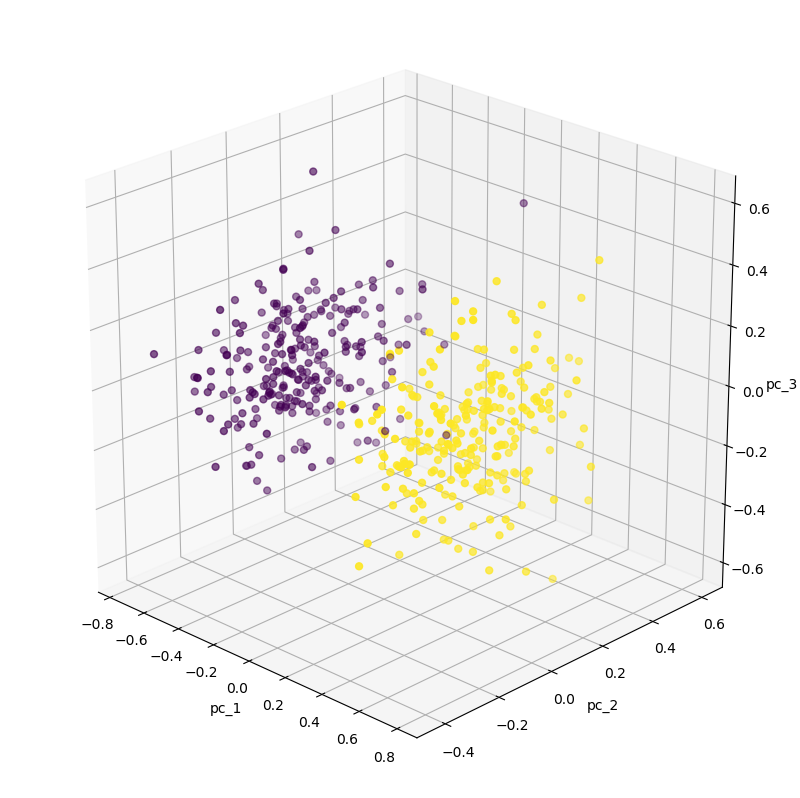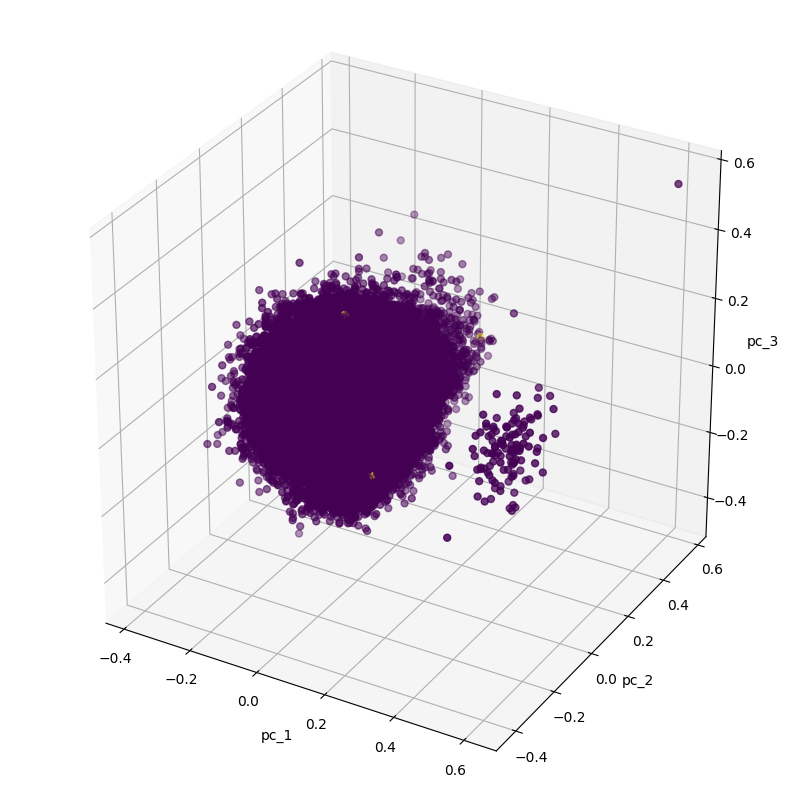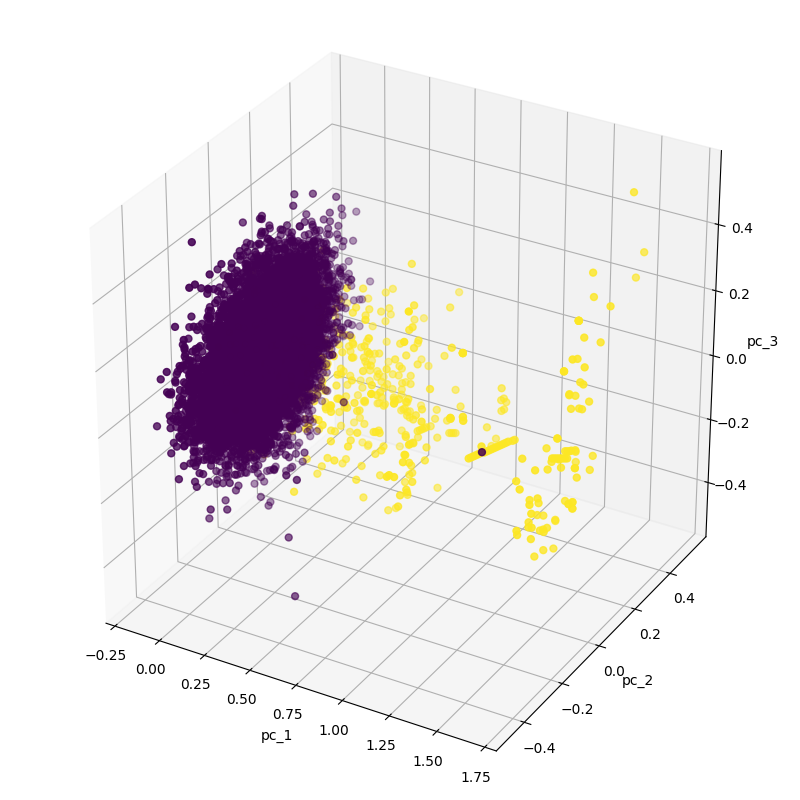1610403000

# Applications of Autoencoders - Anomaly Detection

Autoencoders can be used for anomaly detection by setting limits on the reconstruction error. All ‘good’ data points fall within the acceptable error and any outliers are considered anomalies. This approach can be used for images or other forms of data. This video tutorial explains the process using a synthetic dataset stored in a csv file.

The code from this video is available at: https://github.com/bnsreenu/python_for_microscopists

#python #data-science

## Buddha Community1618310820

## These Tips Will Help You Step Up Anomaly Detection Using ML

In this article, you will learn a couple of Machine Learning-Based Approaches for Anomaly Detection and then show how to apply one of these approaches to solve a specific use case for anomaly detection (Credit Fraud detection) in part two.

A common need when you analyzing real-world data-sets is determining which data point stand out as being different from all other data points. Such data points are known as anomalies, and the goal of anomaly detection (also known as outlier detection) is to determine all such data points in a data-driven fashion. Anomalies can be caused by errors in the data but sometimes are indicative of a new, previously unknown, underlying process.

#machine-learning #machine-learning-algorithms #anomaly-detection #detecting-data-anomalies #data-anomalies #machine-learning-use-cases #artificial-intelligence #fraud-detection1618128600

## Credit Card Fraud Detection via Machine Learning: A Case Study

This is the second and last part of my series which focuses on Anomaly Detection using Machine Learning. If you haven’t already, I recommend you read my first article here which will introduce you to Anomaly Detection and its applications in the business world.

In this article, I will take you through a case study focus on Credit Card Fraud Detection. It is important that credit card companies are able to recognize fraudulent credit card transactions so that customers are not charged for items that they did not purchase. So the main task is to identify fraudulent credit card transactions by using Machine learning. We are going to use a Python library called PyOD which is specifically developed for anomaly detection purposes.

#machine-learning #anomaly-detection #data-anomalies #detecting-data-anomalies #fraud-detection #fraud-detector #data-science #machine-learning-tutorials1596003960

## Anomaly detection with Autoencoders

A nomalies in systems occur rarely. The validation layers stand guard over correctness by catching them out and eliminating them from the process. A cash withdrawal request in a place that is unusual for the card owner or a sensor reading that exceeds the norms can be verified based on profiles or historical data. However, what happens when the event does not differ from the norm at first glance?

# Multidimensional nature of events

Anomalies are not easy to detect. It is often the case that the values of the adopted characteristics subtly deviate from the correct distribution, or the deviation from the norm is only noticeable after taking into account a series of events and time characteristics. In such cases, the standard approach is to analyze the characteristics in terms of e.g. their mutual correlation.

Mirek Mamczur elaborated on this very well in his post.

For our needs, we will generate an artificial data set where one of the classes will be considered anomalies. The events will have 15 characteristics and they will be clustered fairly close together with a standard deviation of 1.75.

``````import matplotlib.pyplot as plt
import pandas as pd
import numpy as np
from mpl_toolkits.mplot3d import Axes3D
from sklearn import datasets, decomposition, preprocessing

x, y = datasets.make_blobs(
n_samples=500,
n_features=15,
centers=2,
center_box=(-4.0, 4.0),
cluster_std=1.75,
random_state=42)

x = preprocessing.MinMaxScaler().fit_transform(x)
pca = decomposition.PCA(n_components=3)
pca_result = pca.fit_transform(x)
print(pca.explained_variance_ratio_)

pca_df = pd.DataFrame(data=pca_result, columns=['pc_1', 'pc_2', 'pc_3'])
pca_df = pd.concat([pca_df, pd.DataFrame({'label': y})], axis=1)

ax = Axes3D(plt.figure(figsize=(8, 8)))
ax.scatter(xs=pca_df['pc_1'], ys=pca_df['pc_2'], zs=pca_df['pc_3'], c=pca_df['label'], s=25)
ax.set_xlabel("pc_1")
ax.set_ylabel("pc_2")
ax.set_zlabel("pc_3")
plt.show()
view raw
pca.py hosted with ❤ by GitHub
``````

To get the model to make an effort, we can force closer data generation by reducing [center_box].#keras #autoencoder #anomaly-detection #machine-learning #artificial-intelligence #deep learning1604230740

## What is an Anomaly?

An anomaly by definition is something that deviates from what is standard, normal, or expected.

When dealing with datasets on a binary classification problem, we usually deal with a balanced dataset. This ensures that the model picks up the right features to learn. Now, what happens if you have very little data belonging to one class, and almost all data points belong to another class?

In such a case, we consider one classification to be the ‘normal’, and the sparse data points as a deviation from the ‘normal’ classification points.

For example, you lock your house every day twice, at 11 AM before going to the office and 10 PM before sleeping. In case a lock is opened at 2 AM, this would be considered abnormal behavior. Anomaly detection means predicting these instances and is used for Intrusion Detection, Fraud Detection, health monitoring, etc.

In this article, I show you how to use pycaret on a dataset for anomaly detection.

## What is PyCaret?

PyCaret is an open-source, low-code machine learning library in Python that aims to reduce the cycle time from hypothesis to insights. It is well suited for seasoned data scientists who want to increase the productivity of their ML experiments by using PyCaret in their workflows or for citizen data scientists and those **new to data science **with little or no background in coding. PyCaret allows you to go from preparing your data to deploying your model within seconds using your choice of notebook environment.

So, simply put, pycaret makes it super easy for you to visualize and train a model on your datasets within 3 lines of code!

So let’s dive in!

#anomaly-detection #machine-learning #anomaly #fraud-detection #pycaret1595578200

## Applying Anomaly Detection with Autoencoders to Fraud Detection

I recently read an article called Anomaly Detection with Autoencoders. The article was based on generated data, so it sounded like a good idea to apply this idea to a real-world fraud detection task and validate it.

I decided to use Credit Card Fraud Dataset From Kaggle*:

The datasets contains transactions made by credit cards in September 2013 by european cardholders.

This dataset presents transactions that occurred in two days, where we have 492 frauds out of 284,807 transactions. The dataset is highly unbalanced, the positive class (frauds) account for 0.172% of all transactions.

It is a very unbalanced dataset and a good candidate to identify fraud through anomalies.

We are going to do a smaller plot after decreasing our dimensions from 30 to 3 with Principal Component Analysis. This data has 32 columns where the first column is the time index, 29 unknown features, 1 transaction amount, and 1 class. I will ignore the time index since it is not stationary.

``````def show_pca_df(df):
x = df[df.columns[1:30]].to_numpy()
y = df[df.columns].to_numpy()

x = preprocessing.MinMaxScaler().fit_transform(x)
pca = decomposition.PCA(n_components=3)
pca_result = pca.fit_transform(x)
print(pca.explained_variance_ratio_)

pca_df = pd.DataFrame(data=pca_result, columns=['pc_1', 'pc_2', 'pc_3'])
pca_df = pd.concat([pca_df, pd.DataFrame({'label': y})], axis=1)

ax = Axes3D(plt.figure(figsize=(8, 8)))
ax.scatter(xs=pca_df['pc_1'], ys=pca_df['pc_2'], zs=pca_df['pc_3'], c=pca_df['label'], s=25)
ax.set_xlabel("pc_1")
ax.set_ylabel("pc_2")
ax.set_zlabel("pc_3")
plt.show()

show_pca_df(df)
view raw
anomaly_detection_part1_1.py hosted with ❤ by GitHub
``````Your first reaction could be that there are two clusters and this would be an easy task but fraud data is yellow points! There are three visible yellow points in the large cluster. So let’s subsample the normal data while keeping the number of fraud data.

``````df_anomaly = df[df[df.columns] > 0]
df_normal = df[df[df.columns] == 0].sample(n=df_anomaly.size, random_state=1, axis='index')
df = pd.concat([ df_anomaly, df_normal])

show_pca_df(df)
view raw
anomaly_detection_part1_2.py hosted with ❤ by GitHub
``````#keras #anomaly-detection #deep-learning #tensorflow #fraud-detection #deep learning